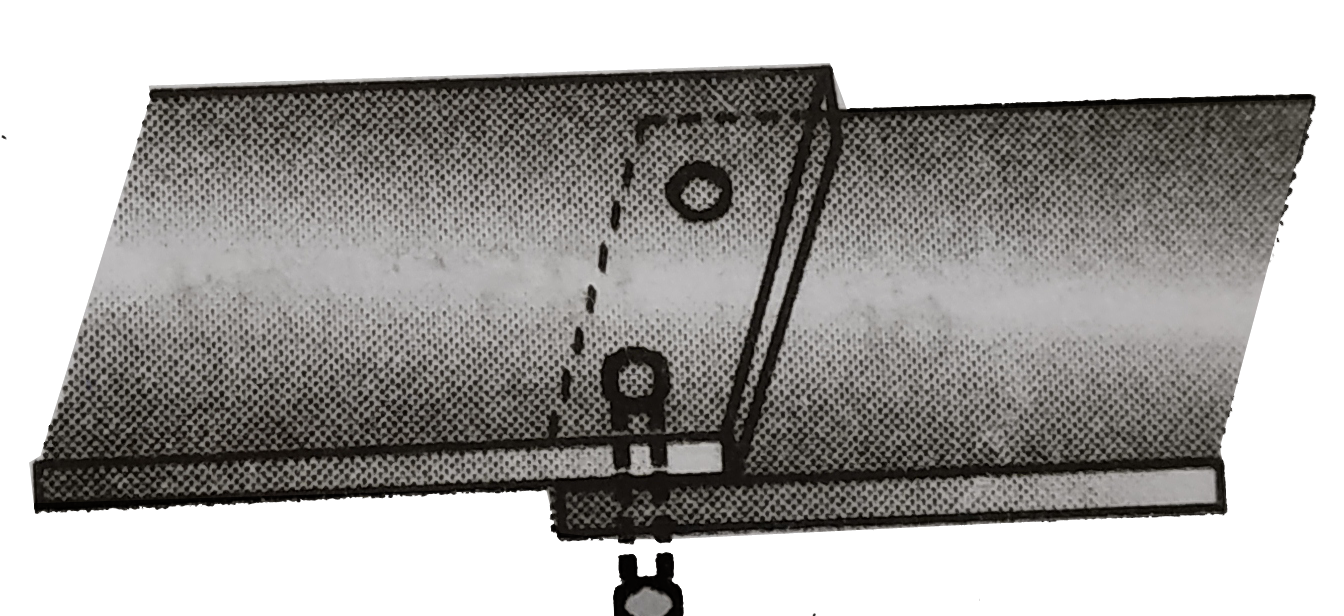# Two long metallic strips are joined together by two rivets each of radius 2 mm. Each rivet can withstand a maximum shearing stress of 1.5 xx 10^(9)

236 views
in Physics
closed
Two long metallic strips are joined together by two rivets each of radius 2 mm. Each rivet can withstand a maximum shearing stress of 1.5 xx 10^(9) N//m^(2). Assuming that each rivet shares the stretching load equally, the maximum tensile force the strip can exert without rupture is
A. 1.88xx10^(4)N
B. 3.8xx10^(4)N
C. 6xx10^(7)N
D. 3xx10^(4)N

by (87.1k points)
selected

Let the maximum tensile force that each river can experience be F, then maximum shearing stress on each river is
sigma=F/A=1.5xx10^(9)
A=pir^(2), where r=2xx10^(-3)m
Required force is 2F`.